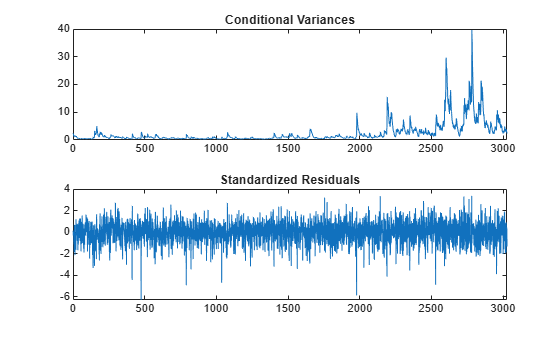Documentation

## Estimate Conditional Mean and Variance Models

This example shows how to estimate a composite conditional mean and variance model using `estimate`.

### Load the Data and Specify the Model.

Load the NASDAQ data included with the toolbox. Convert the daily close composite index series to a return series. For numerical stability, convert the returns to percentage returns. Specify an AR(1) and GARCH(1,1) composite model. This is a model of the form

`${r}_{t}=c+{\varphi }_{1}{r}_{t-1}+{\epsilon }_{t},$`

where ${\epsilon }_{t}={\sigma }_{t}{z}_{t}$,

`${\sigma }_{t}^{2}=\kappa +{\gamma }_{1}{\sigma }_{t-1}^{2}+{\alpha }_{1}{\epsilon }_{t-1}^{2},$`

and ${z}_{t}$ is an independent and identically distributed standardized Gaussian process.

```load Data_EquityIdx nasdaq = DataTable.NASDAQ; r = 100*price2ret(nasdaq); T = length(r); Mdl = arima('ARLags',1,'Variance',garch(1,1))```
```Mdl = arima with properties: Description: "ARIMA(1,0,0) Model (Gaussian Distribution)" Distribution: Name = "Gaussian" P: 1 D: 0 Q: 0 Constant: NaN AR: {NaN} at lag  SAR: {} MA: {} SMA: {} Seasonality: 0 Beta: [1×0] Variance: [GARCH(1,1) Model] ```

### Estimate the Model Parameters Without Using Presample Data.

Fit the model, `Mdl`, to the return series, `r`, using `estimate`. Use the presample observations that `estimate` automatically generates.

`EstMdl = estimate(Mdl,r);`
``` ARIMA(1,0,0) Model (Gaussian Distribution): Value StandardError TStatistic PValue ________ _____________ __________ __________ Constant 0.072632 0.018047 4.0245 5.7088e-05 AR{1} 0.13816 0.019893 6.945 3.7848e-12 GARCH(1,1) Conditional Variance Model (Gaussian Distribution): Value StandardError TStatistic PValue ________ _____________ __________ __________ Constant 0.022377 0.0033201 6.7399 1.5852e-11 GARCH{1} 0.87312 0.0091019 95.928 0 ARCH{1} 0.11865 0.008717 13.611 3.434e-42 ```

The estimation display shows the five estimated parameters and their corresponding standard errors (the AR(1) conditional mean model has two parameters, and the GARCH(1,1) conditional variance model has three parameters).

The fitted model (`EstMdl`) is

`${r}_{t}=0.073+0.138{r}_{t-1}+{\epsilon }_{t},$`

where ${\epsilon }_{t}={\sigma }_{t}{z}_{t}$ and

`${\sigma }_{t}^{2}=0.022+0.873{\sigma }_{t-1}^{2}+0.119{\epsilon }_{t-1}^{2}.$`

All t statistics are greater than two, suggesting all parameters are statistically significant.

### Infer the Conditional Variances and Residuals.

Infer and plot the conditional variances and standardized residuals. Also output the loglikelihood objective function value.

```[res,v,logL] = infer(EstMdl,r); figure subplot(2,1,1) plot(v) xlim([0,T]) title('Conditional Variance') subplot(2,1,2) plot(res./sqrt(v)) xlim([0,T]) title('Standardized Residuals')```The conditional variances increase after observation 2000. This corresponds to the increased volatility seen in the original return series.

The standardized residuals have more large values (larger than 2 or 3 in absolute value) than expected under a standard normal distribution. This suggests a Student's t distribution might be more appropriate for the innovation distribution.

### Fit a Model With a t-Innovation Distribution.

Modify the model so that it has a Student's t-innovation distribution. Fit the modified model to the NASDAQ return series. Specify an initial value for the variance model constant term.

```MdlT = Mdl; MdlT.Distribution = 't'; EstMdlT = estimate(MdlT,r,'Variance0',{'Constant0',0.001});```
``` ARIMA(1,0,0) Model (t Distribution): Value StandardError TStatistic PValue ________ _____________ __________ __________ Constant 0.093488 0.016694 5.6002 2.1414e-08 AR{1} 0.13911 0.018857 7.3771 1.6175e-13 DoF 7.4776 0.88262 8.472 2.4128e-17 GARCH(1,1) Conditional Variance Model (t Distribution): Value StandardError TStatistic PValue ________ _____________ __________ __________ Constant 0.011246 0.0036305 3.0976 0.001951 GARCH{1} 0.90766 0.010516 86.315 0 ARCH{1} 0.089897 0.010835 8.2966 1.071e-16 DoF 7.4776 0.88262 8.472 2.4128e-17 ```

The coefficient estimates change slightly when the t distribution is used for the innovations. The second model fit (`EstMdlT`) has one additional parameter estimate, the t distribution degrees of freedom. The estimated degrees of freedom are relatively small (about 8), indicating significant departure from normality.

### Compare the Model Fits.

Compare the two model fits (Gaussian and t-innovation distribution) using the Akaike information criterion (AIC) and Bayesian information criterion (BIC). First, obtain the loglikelihood objective function value for the second fit.

```[resT,vT,logLT] = infer(EstMdlT,r); [aic,bic] = aicbic([logL,logLT],[5,6],T)```
```aic = 1×2 103 × 9.4929 9.3807 ```
```bic = 1×2 103 × 9.5230 9.4168 ```

The second model has six parameters compared to five in the first model (because of the t distribution degrees of freedom). Despite this, both information criteria favor the model with the Student's t distribution. The AIC and BIC values are smaller for the t innovation distribution.